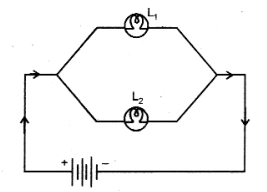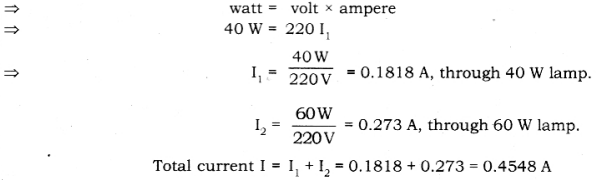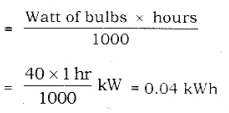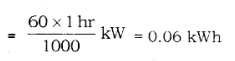# Two lamps, one rated 40 W at 220 V and the other 60 W at 220 V, are connected in parallel

Two lamps, one rated 40 W at 220 V and the other 60 W at 220 V, are connected in parallel to the electric supply at 220 V.

1. Draw a circuit diagram to show the connections.
2. Calculate the current drawn from the electric supply.
3. Calculate the total energy consumed by the two lamps together when they operate for one hour.
1.2. P = VI3. Energy consumed by 40 W bulbEnergy consumed by 60 W bulbTotal energy consumed by 60 W bulb = 0.06 kWh,